# CHEMICAL KINETICS: INTRODUCTION TO RATE OF REACTION

Chemical kinetics deals with the rates of chemical reactions i.e., how fast a reaction occurs? It is observed that some reactions occur within a fraction of second, whereas some reactions take years together for completion.

Consider the following chemical changes, which occur at different speeds.

i) Rusting of iron, which involves the oxidation of iron, occurs comparatively at slower rate. Depending on the conditions, it may take days to months or years together to undergo complete change. It is a very slow reaction.

ii) Digestion of food involves very simple to complex chemical and physical changes. Majorly it is the breaking down of bigger molecules into smaller molecules by hydrolysis process. Usually the food is digested in 3 to 4 hours time. It is indeed a reaction with medium speed.

iii) Esterification of acetic acid by ethyl alcohol in presence of acid is also another example for reaction with medium speed since it takes about 24 hours of time to reach equilibrium.

iv) The formation of a white precipitate of silver chloride, AgCl from a solution containing chloride ions, Cl- by adding aqueous solution of AgNO3 occurs in a fraction of second. It is a very fast reaction.

In the present topic - “chemical kinetics” we deal with the answers to the questions like:

i) Why do some reactions occur faster and some occur at slower rates?;

ii) What are the factors affecting the speed?;

iii) How can we speed up a reaction?;

iv) By what mechanism, a chemical reaction occurs?

The study of chemical kinetics deals with the qualitative and quantitative study of:

i) the rates of reactions,

ii) the factors affecting rate of reaction and

iii) the mechanisms of reactions.

It also explains why some of the thermodynamically feasible reactions occur slowly; or do not occur unless initiated by applying suitable conditions. For example, burning of wood is a spontaneous or feasible process according to thermodynamic laws. But wood cannot burn itself. It starts burning only after igniting it.

So now the question is: What is exactly speed or rate of a reaction? How do we express it?

## RATE OF REACTION

The rate of a reaction can be expressed as:

1) average rate

2) instantaneous rate

Average rate: The change in molar concentration of either reactants or products in unit time is called as average rate.Let ‘Δc’ is the decrease in concentration of reactants in a small interval of time ‘Δt’ then:(negative sign is used to make the rate positive, since the concentration of reactants decreases with time)

If ‘Δx’ is the increase in concentration of products in a small interval of time ‘Δt’ then: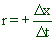( positive sign is used since there is increase in the concentration of products during the reaction )

During the reaction, the concentration of reactants decreases, whereas the concentration of products increases exponentially until they reach an equilibrium position (see the graphs below).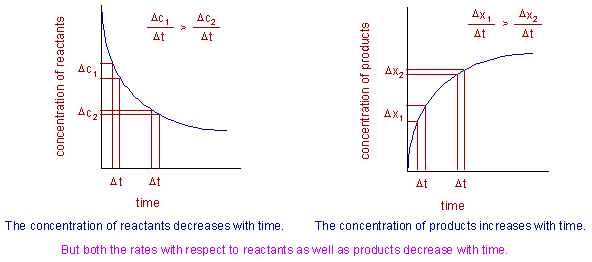That means the rate of the reaction is not same at all time intervals during the reaction. The rate of the reaction decreases exponentially with time as illustrated below. Notice that for same time intervals, Δt the change in concentration is not same.

Instantaneous rate: The change in molar concentration of either reactants or products at an instant of time (or in infinitesimally small interval of time) is called as instantaneous rate.Where

dc = change in the concentration of reactant in infinitesimally small interval of time ‘dt’

dx = change in the concentration of product in infinitesimally small interval of time ‘dt’

Graphically, the instantaneous rate of the reaction can be calculated by measuring the slope of the tangent drawn at a given point on the curve plotted between concentration versus time as shown below: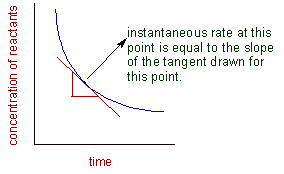For a general reaction,

pP + qQ --------> rR + sS

the rate of reaction can be expressed as: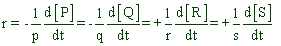Where the small letters: p, q, r & s represent the stoichiometric coefficients and the capital letters in square brackets: [P], [Q], [R] & [S] indicate the molar concentrations of P, Q, R & S.

Thus it is possible to write a general equation for the rate as follows:Where:

ni = stoichiometric coefficient of ‘i’ in the balanced equation

ci = concentration of ‘i’

A confusion about rate: The above general equation for the rate may be confusing since the ni value may vary if we write the balanced equation in other way.

E.g. The dissociation of hydrogen peroxide can be balanced in two different ways as: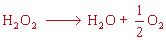(or)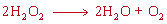According to the first version, the rate can be expressed as:But according to the second version, the rate can be expressed as:The rate expressed for second version has half the value of the rate expressed for the first version.

But the individual values of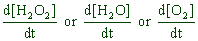remain same irrespective of the version of balanced equation (however they are not equal; see below). Therefore in other way, the rate can be defined simply, without considering the balanced equation, as: the change in concentration of either reactant or product at an instant of time.This is actually what we are measuring experimentally as rate. However, in this way, the rate with respect to different species will have different values.

i.e.,So be careful and do not confuse yourself. It is not the problem with us but with the way of defining the rate.

The rate of a reaction is expressed in the units of (concentration) (time)-1 like: M sec-1 (or) M min-1 (or) mol.L-1 sec-1 (or) mol.L-1 min-1

For gases, the activities are expressed in terms of partial pressures. Therefore the unit for the rate of reaction may be atm.s-1 or atm min-1.

 Chemical kinetics - TOC Collision theory of chemical kinetics >